# Electric Motor Horsepower Calculator

The term “horsepower” was derived by Scottish Engineer James Watt in the 18th century to compare the output of steam engines with the power of horses. Ever since, the term has been expanded to describe the output power in other systems, such as electric motors.

But when it comes to electric motor horsepower calculations, there are several things that every engineer must know. This article covers electric motor horsepower design calculations. It also covers calculations on motor efficiency and full load amperage rating.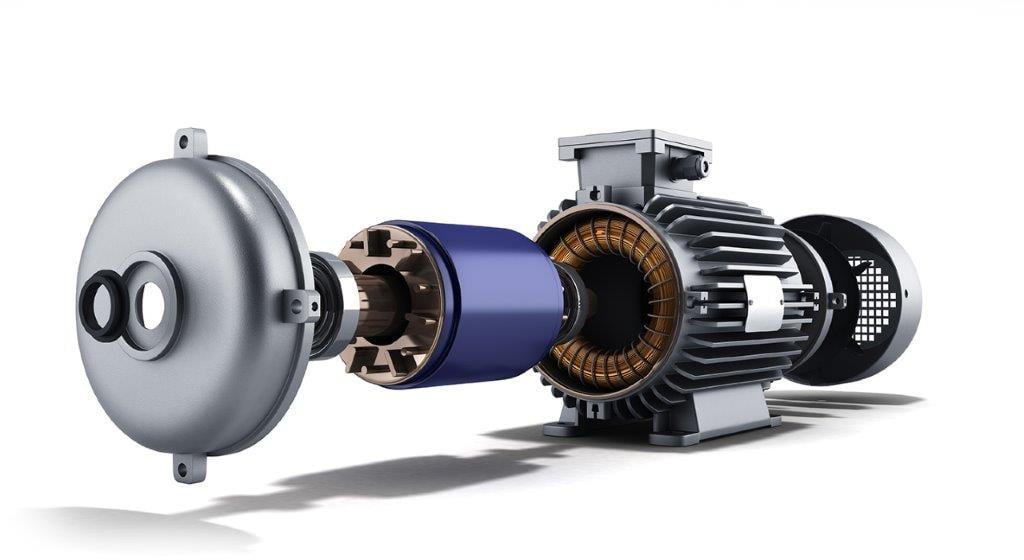There are several things engineers must know about electric motor horsepower calculations

## Motor Horsepower Calculator

Electric motors convert electrical energy to mechanical energy output in the form of shaft rotation. This mechanical output can be calculated using: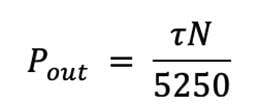Where:

Pout: Output power (horsepower)

T=Torque (lb-ft)

N=Rotational Speed (revolutions per minute)

The electric power input for single-phase electric motors can be estimated using: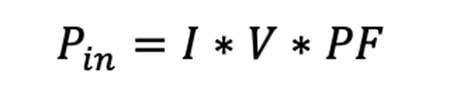The electric power input for three-phase electric motors can be estimated using: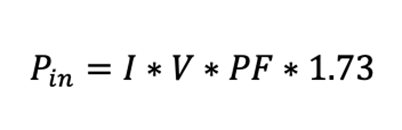Where:

I=Electric current (line)

V=Line voltage (V)

PF=Power factor

Pin=Power input (Watt)

## Motor Efficiency Calculator

In an ideal electric motor, the total electric power input is converted to mechanical power output. However, this is not the case with the real-life operation of electric motors. Losses due to heat and friction make the motor power output less than electric power input. Electric motor efficiency simply describes how much of the electrical power input the motor converts to power output.

Motor efficiency can be calculated using: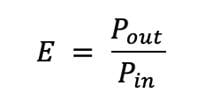## Motor efficiency calculation for 1-phase motors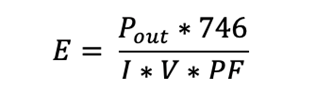## Motor efficiency calculation for 3-phase motors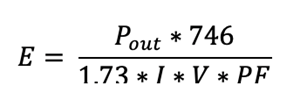Where:

I=Motor amperage

V=Voltage

PF=Power factor

Pout=motor power output (hp)

## Electric Motor Full Load Amperage Calculation

The full load amperage is the amount of current the electric motor draws while it is producing its rated output horsepower at its rated voltage. Most electric motor manufacturers specify the full load amps rating on the nameplate of their electric motors. For example, the image below shows the nameplate of a typical three-phase induction motor. The full load amperage of this motor is 0.97A at 400V.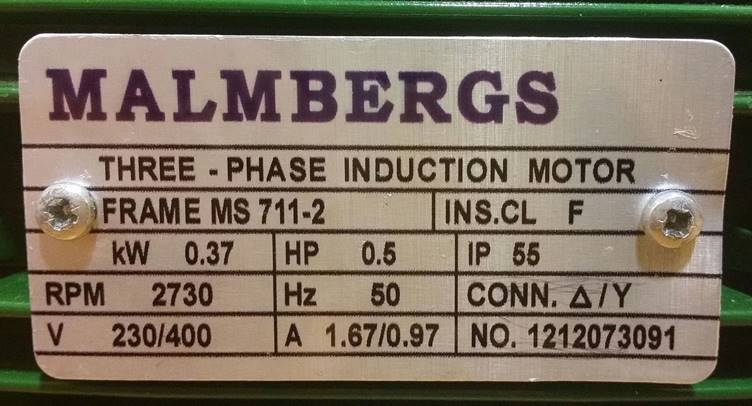Figure 2. Nameplate of a typical three-phase induction motor

Source: [Olgailga2/Public domain]

Engineers can also calculate the full load amperage (also called FLA or amps) using the sets of equations in the following sections.

## 1-phase motor FLA calculator

The full load amperage for single-phase motors can be calculated using: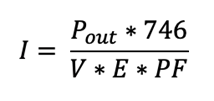## 3-phase motor amperage calculator

The full load amperage for three-phase motors can be calculated using: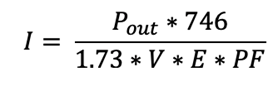Where:

P=Mechanical power output

PF=Power factor

V=Line voltage

E=The efficiency of the motor

## Determining Motor Overload using motor horsepower calculations

Electric motor overload or overcurrent occurs when an electric motor is under excessive load. This is usually caused when the amount of current flow in the motor windings exceeds the current that the motor is designed to carry. In simpler terms, an electric motor is overloaded if the calculated power output in horsepower is greater than the nameplate rating.

For example, consider a 1 hp single-phase motor with a power factor of 0.75 and an efficiency of 85% and is used to drive a pump. If the engineer determines the voltage and current values to be 124V and 12A, respectively, then the following calculations can be used to determine if the motor is overloaded.

First, compute the electric power input: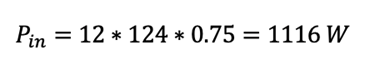In horsepower unit, this electric power input value is 1116/746=1.496 hp

Now since the electric motor efficiency is known to be 95%, the motor power output can simply be calculated using the efficiency formula: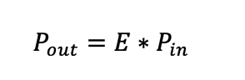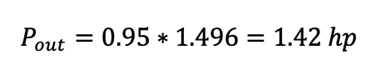Now since the motor horsepower rating was stated to be 1 Hp, the calculated motor power output exceeds this horsepower rating. This means the motor is overloaded. And if the electric motor is left at this operating condition for a long period, it causes the motor windings to overheat and damages the motor components.

Overload protection devices help to protect the motor from damages resulting from motor overload. These devices monitor the current flowing in the motor circuit and trip if this current rises above a certain current limit. Engineers can size overload protection devices and determine this current limit by using the motor full load amps found on the motor nameplate.

According to the NEC 430.32 standards, engineers should size an overload device for continuous duty motors marked with a service life of 1.15 or greater and a temperature rise of 40°C at no more than 125% of the motor full load amps rating. NEC standard also states that engineers should size the overload protection device at 115% of the motor FLA for all other motors.

## Conclusion

While this article presents helpful tips about electric motor horsepower calculations, there are still several things an engineer must consider when specifying electric motors. For example, engineers still need to determine the winding size, consider the operational environment, and plan for available power supply. As such, engineers are advised to reach out to electric motor manufacturers to discuss their application requirements.